# ISEE Middle Level Math : How to find the perimeter of square

## Example Questions

1 3 Next →

### Example Question #21 : Squares

Find the perimeter of a square with a width of 9in.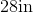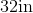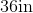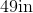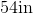Explanation:

To find the perimeter of a square, we will use the following formula: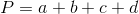where a, b, c, and d are the lengths of the sides of the square.

Now, we know the width of the square is 9in.  Because it is a square, all sides are equal. Therefore, all sides are 9in.  So, we can substitute.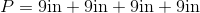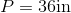### Example Question #112 : Plane Geometry

Find the perimeter of a square with a width of 9cm.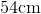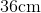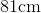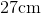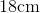Explanation:

To find the perimeter of a square, we will use the following formula:where a, b, c, and d are the lengths of the sides of the square.

Now, we know the width of the square is 9cm.  Because it is a square, all sides are equal.  Therefore, all sides are 9cm.  So, we can substitute.  We get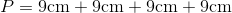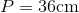### Example Question #22 : Squares

Find the perimeter of a square with a length of 6in.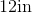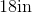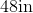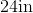Explanation:

To find the perimeter of a square, we will use the following formula:where a, b, c, and d are the lengths of the sides of the square.

Now, we know the length of the square is 6in.  Because it is a square, all sides are equal.  Therefore, all sides are 6in.  So, we can substitute.  We get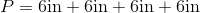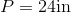### Example Question #111 : Plane Geometry

Find the perimeter of a square with a length of 14cm.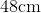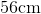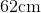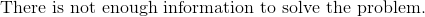Explanation:

To find the perimeter of a square, we will use the following formula:where a, b, c, and d are the lengths of the sides of the square.

Now, we know the width of the square is 14cm.  Because it is a square, all sides are equal.  Therefore, all sides are 14cm.  So, we get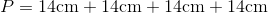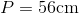### Example Question #21 : Quadrilaterals

Find the perimeter of a square that has a length of 13in.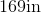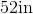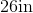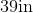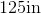Explanation:

To find the perimeter of a square, we will use the following formula:where a, b, c, and d are the lengths of the sides of the square.

Now, we know the length of the square is 13in.  Because it is a square, all sides are equal.  Therefore, all sides are 13in.

Knowing this, we can substitute into the formula.  We get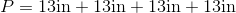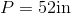1 3 Next →

### All ISEE Middle Level Math Resources